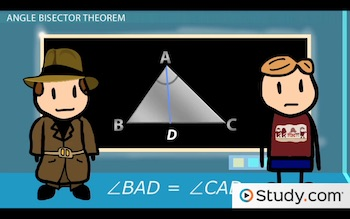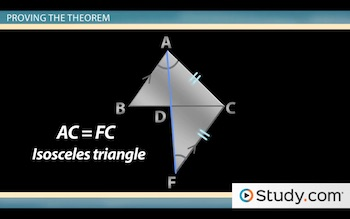# Angle Bisector Theorem: Proof and Example

An error occurred trying to load this video.

Try refreshing the page, or contact customer support.

Coming up next: Congruency of Right Triangles: Definition of LA and LL Theorems

### You're on a roll. Keep up the good work!

Replay
Your next lesson will play in 10 seconds
• 0:03 Angle Bisector Theorem
• 1:24 Proving the Theorem
• 4:03 Examples
• 5:40 Lesson Summary
Save Save

Want to watch this again later?

Timeline
Autoplay
Autoplay
Speed Speed

#### Recommended Lessons and Courses for You

Lesson Transcript
Instructor: Jeff Calareso

Jeff teaches high school English, math and other subjects. He has a master's degree in writing and literature.

The angle bisector theorem sounds almost too good to be true. In this lesson, we set out to prove the theorem and then look at a few examples of how it's used.

## Angle Bisector Theorem

It's time to play detective. There's a theorem involving angle bisectors and triangles that sounds a little fishy. Let's do some investigating and see what we can find.

Here's triangle ABC:This was always a good triangle, never getting into trouble. One day, it got itself mixed up with an angle bisector. That's right - this line from A to BC. We label the point where the angle bisector hits BC as point D. Since it's an angle bisector, it bisects the angle from which it's drawn.

That means angle BAD is congruent to angle CAD. Everything seemed great for the triangle...at first. It was said that there was a theorem we could use - the angle bisector theorem. But it sounded too good to be true. The angle bisector theorem states that an angle bisector divides the opposite side of a triangle into two segments that are proportional to the triangle's other two sides. In other words, AB/BD = AC/CD.

How could that be true? The angle bisector makes two smaller triangles that are proportional to each other. Somebody had to prove the theorem, and that's where we come in.

## Proving the Theorem

We all wanted the theorem to be true. But is it? We'll need to get our hands a little dirty to find out. To start, let's extend our angle bisector, AD, out a little further. Now let's add a line that's parallel to AB that hits point C and crosses our extended bisector. We'll label this point F.

We can hardly recognize poor old triangle ABC anymore. It's sad, I know. But this is what the triangle wanted. And, trust me, if we want to prove that AB/BD = AC/CD, we need to break some eggs. Well, by breaking eggs, I mean adding lines and stuff.

Okay, time to start putting the pieces together. We know angle BAD equals angle DFC. Why? If AB and FC are parallel, then these are alternate interior angles, and alternate interior angles are equal. That means that angle DFC also equals angle CAD. Remember, BAD and CAD are equal because of the angle bisector.

If we look at triangle ACF below, we have two equal angles, which makes this an isosceles triangle. So, AC = FC. I told you we'd have to break some eggs to solve this case. And we're not there yet. But we're close.Let's look at two more angles. Angle ADB is congruent to angle CDF. Why? They're vertical angles. And vertical angles are congruent.

Now look at those two small triangles above - ADB and FDC - where we have two congruent angles. We want to be sure to match the right angles - A to F, D to D and B to C. That means that we can state that triangle ADB is similar to triangle FDC because of the angle-angle similarity. Similar triangles are in proportion to one another. Yep, the dots are all connecting now, aren't they? So we can say that AB/BD = FC/CD.

To unlock this lesson you must be a Study.com Member.

### Register to view this lesson

Are you a student or a teacher?

#### See for yourself why 30 million people use Study.com

##### Become a Study.com member and start learning now.
Back
What teachers are saying about Study.com

### Earning College Credit

Did you know… We have over 200 college courses that prepare you to earn credit by exam that is accepted by over 1,500 colleges and universities. You can test out of the first two years of college and save thousands off your degree. Anyone can earn credit-by-exam regardless of age or education level.